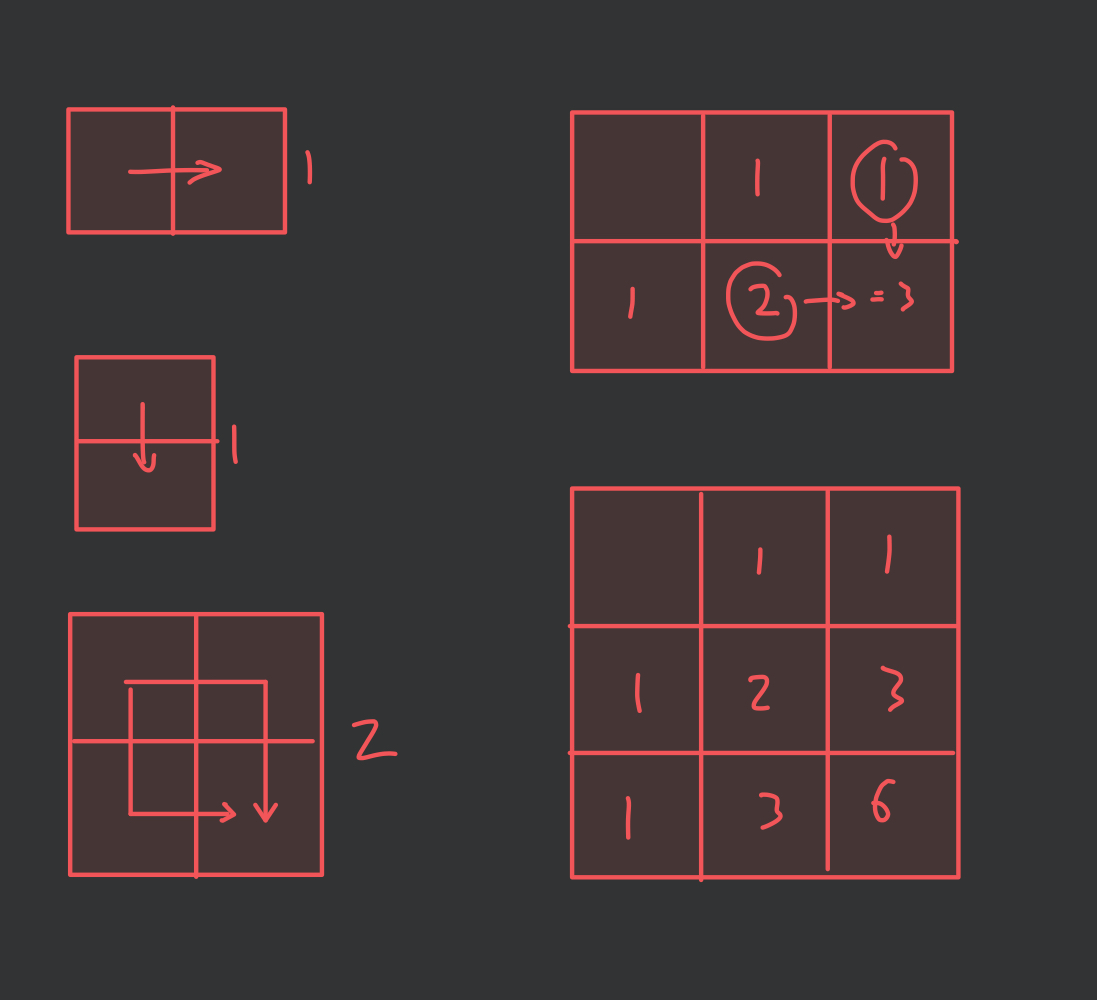# 2d matrix ways

🏠

Calculate the number of ways of traversing a matrix from 0,0 to n,m by only moving to the right or down.1from test_framework import generic_test
2from functools import lru_cache
3
4
5def number_of_ways(n: int, m: int) -> int:
6    @lru_cache(None)
7    def dp(w, h):
8        if w == 1 or h == 1:
9            return 1
10        return dp(w - 1, h) + dp(w, h - 1)
11
12    return dp(n, m)
13
14
15if __name__ == "__main__":
16    exit(
17        generic_test.generic_test_main(
18            "number_of_traversals_matrix.py",
19            "number_of_traversals_matrix.tsv",
20            number_of_ways,
21        )
22    )